# Reducing variance in conversion A/B testing with CUPED

Marton Trencseni - Sat 07 August 2021 - ab-testing

## Simulating many experiments

As with continuous variables, CUPED measures the same lift [in conversion], but with lower variance:

Simulating 10,000 A/B tests, true treatment lift is 0.010...
Traditional    A/B testing, mean lift = 0.010, variance of lift = 0.00030
CUPED adjusted A/B testing, mean lift = 0.010, variance of lift = 0.00019
CUPED lift variance / tradititional lift variance = 0.626 (expected = 0.668)


We can observe the tighter lifts on a histogram: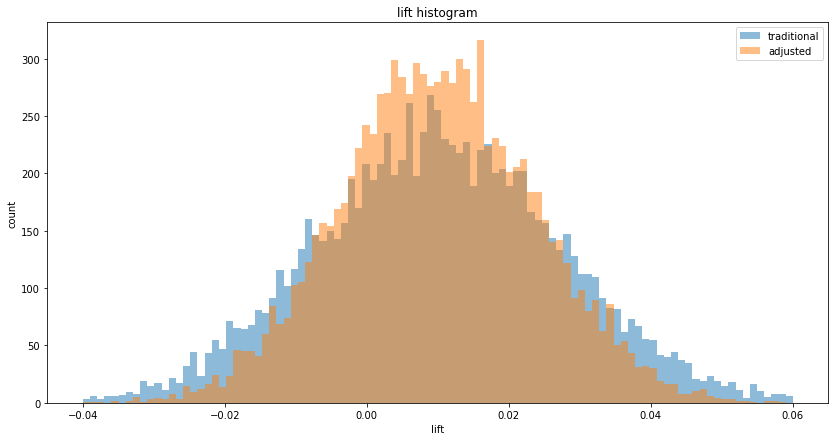As with continuous variables, the p-values decrease: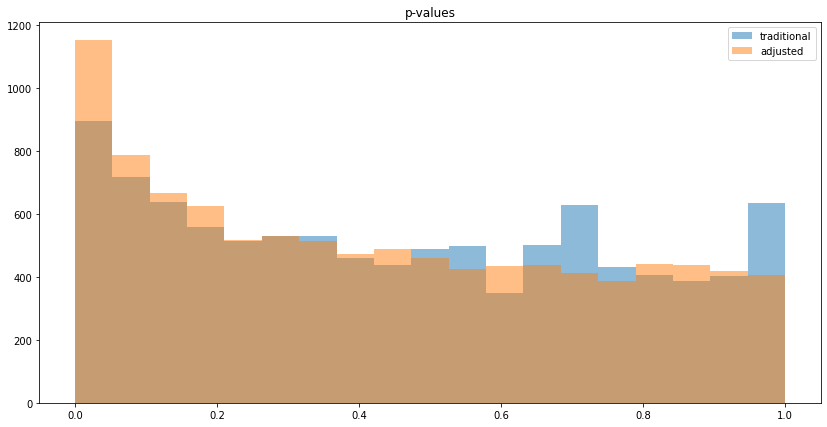As illustrated above, CUPED lifts and p-values are a better estimate with respect to variance, but not in all cases: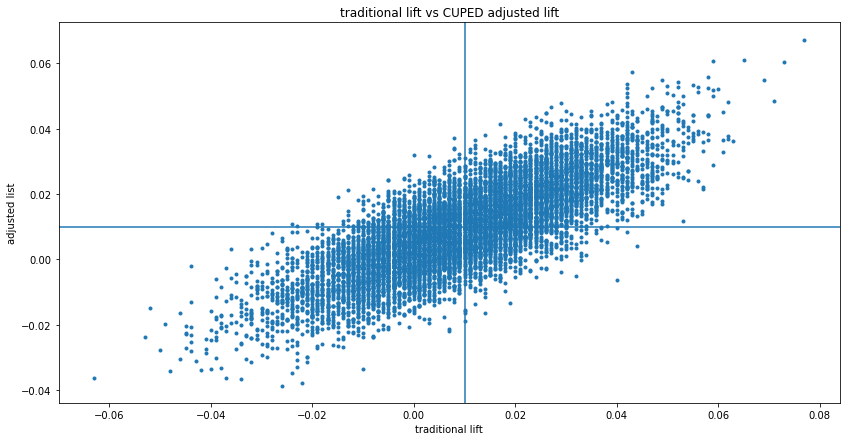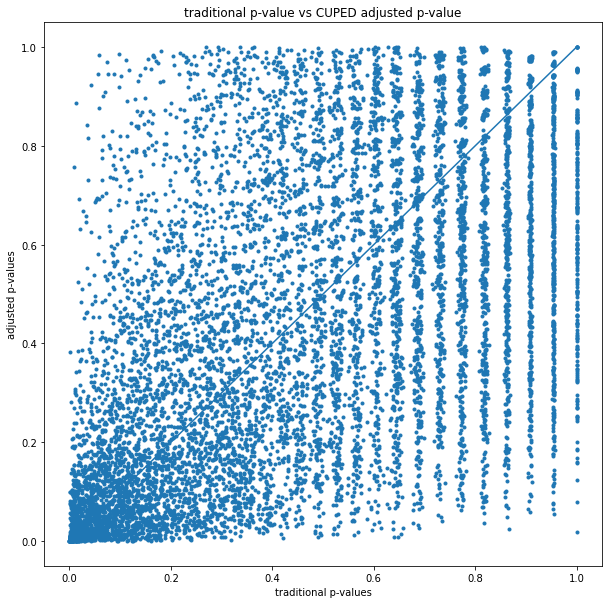## No correlation

The simplest way to generate uncorrelated conversion data is to use random draws independently. In code:

def get_AB_samples_nocorr(before_p, treatment_lift, N):
A_before = [before_p] * N
B_before = [before_p] * N
A_after = [before_p] * N
B_after = [before_p + treatment_lift] * N
return lmd(A_before), lmd(B_before), lmd(A_after), lmd(B_after)


Checking conditional probabilities:

P(after = 1 | before = 0) = 0.10
P(after = 0 | before = 0) = 0.90
P(after = 1 | before = 1) = 0.10
P(after = 0 | before = 1) = 0.90


It's uncorrelated, because P(after = 1) = 0.10 in both 0 and 1 before cases, so "after" is independent of "before" (and same for P(after = 0)).

With this generator function, running num_experiments=10,000, we can observe no variance reduction (since "before" and "after" is uncorrelated) on the lift and p-value histograms: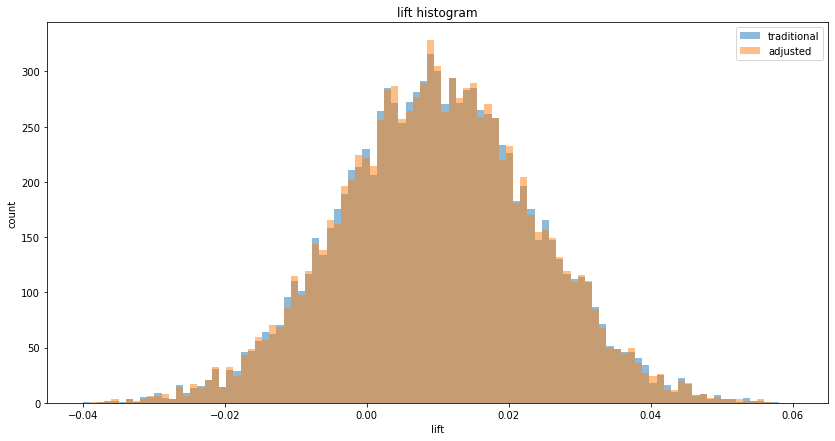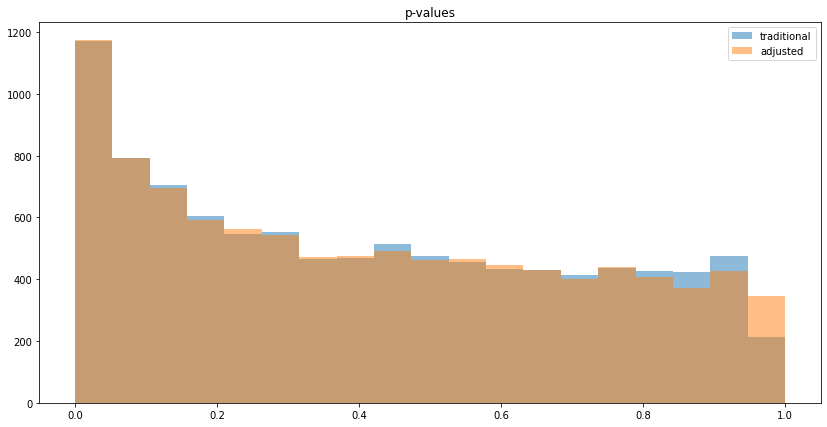## Conclusion

CUPED works for both continuous and binary experimentation outcomes, and reduces variance if "before" and "after" are correlated.